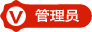#### 程序（VFB教程2-7）2018-2-16 2436

Sub

Sub 是不返回值的过程。它们使用Declare关键字声明，并使用Sub关键字定义。声明过程引入其名称，以便可以调用它，并且过程定义列出了在调用时将执行的代码语句。A sub 简单地通过在程序中的某个地方使用它的名称。

```Sub MyProcedure
Print "the body of MyProcedure"
End Sub```

the body of MyProcedure

Functions

Functions 是将值返回到调用代码中的点的过程。您可以将function 调用视为对某个表达式的评估，就像变量或对象一样。它们使用Declare关键字声明，并使用Function关键字定义。在声明结尾处指定functions 返回的值的类型。

```' introduces and defines a procedure that returns an integer value
Function MyProcedure As Integer
Return 10
End Function

' calls the procedure, and stores its return value in a variable
Dim i As Integer = MyProcedure
Print i```

10

```Sub Procedure (s As String, n As Integer)
Print "The parameters have the values: " & s & " and " & n
End Sub

Procedure "abc", 123```

The parameters have the values: abc and 123

```Sub Procedure (ByVal param As Integer)
param *= 2
Print "The parameter 'param' = " & param
End Sub

Dim arg As Integer = 10
Print "The variable 'arg' before the call = " & arg
Procedure(arg)
Print "The variable 'arg' after the call = " & arg```

The variable 'arg' before the call = 10

The parameter 'param' = 20

The variable 'arg' after the call = 10

```Sub Procedure (ByRef param As Integer)
param *= 2
Print "The parameter 'param' = " & param
End Sub

Dim arg As Integer = 10
Print "The variable 'arg' before the call = " & arg
Procedure(arg)
Print "The variable 'arg' after the call = " & arg```

The variable 'arg' before the call = 10

The parameter 'param' = 20

The variable 'arg' after the call = 20

```Sub f( ByRef i As Integer )
i = 456
End Sub

Dim i As Integer = 123
Dim pi As Integer Ptr = @i

Print i
f( ByVal pi )
Print i```

...指的是Function过程在函数完成时具有值，使得该值可以在表达式中使用或赋值给变量的能力。

```'' Using the name of the function to set the return value and continue executing the function:
Function myfunc1() As Integer
myfunc1 = 1
End Function

'' Using the keyword 'Function' to set the return value and continue executing the function:
Function myfunc2() As Integer
Function = 2
End Function

'' Using the keyword 'Return' to set the return value and immediately exit the function:
Function myfunc3() As Integer
Return 3
End Function```
```'' This program demonstrates a function returning a value.

Declare Function myFunction () As Integer

Dim a As Integer

'Here we take what myFunction returns and add 10.
a = myFunction() + 10

'knowing that myFunction returns 10, we get 10+10=20 and will print 20.
Print a

Function myFunction () As Integer
'Here we tell myFunction to return 10.
Function = 10
End Function```

```Dim Shared i As Integer = 123

Function f( ) ByRef As Integer
Dim pi As Integer Ptr = @i

Function = ByVal pi

'' or, with RETURN it would look like this:
Return ByVal pi
End Function

Print i, f( )```